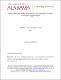## Switching Distributions for Perpendicular Spin-Torque Devices within the Macrospin Approximation2012
##### Abstract

We model “soft” error rates for writing (WSER) and for reading (RSER) for perpendicular spintorque memory devices by solving the Fokker-Planck equation for the probability distribution of the angle that the free layer magnetization makes with the normal to the plane of the film. We obtain: (1) an exact, closed form, analytical expression for the zero-temperature switching time as a function of initial angle; (2) an approximate analytical expression for the exponential decay of the WSER as a function of the time the current is applied; (3) comparison of the approximate analytical expression for the WSER to numerical solutions of the Fokker-Planck equation; (4) an approximate analytical expression for the linear increase in RSER with current applied for reading; (5) comparison of the approximate analytical formula for the RSER to the numerical solution of the Fokker-Planck equation; and (6) confirmation of the accuracy of the FokkerPlanck solutions by comparison with results of direct simulation using the single-macrospin Landau-Lifshitz-Gilbert (LLG) equations with a random fluctuating field in the short-time regime for which the latter is practical.

##### Keywords
Spin-Torque, Fokker-Planck, Switching Distribution, Magnetic Memory, Error Rate, Spin Torque
##### Citation
Butler, W. et al. (2012): Switching Distributions for Perpendicular Spin-Torque Devices within the Macrospin Approximation. IEEE Transactions on Magnetics, 48(12). arXiv:1202.2621v1